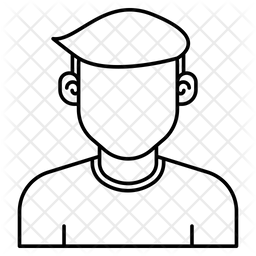# how to determinate ng and nm in wp.rmanova

176 viewsHi,

I am calculating the needed sample size for a new experiment using wp.rmanova function. The experiment is a fully crossed within-subject 2x2 design. I have two factors, and each factor has two levels. It is a signal detection type of experiment. For each condition, it has 180 trials. So, total 180 * 4 = 720 trials for each participant.

I am confused about what the inputs for ng and nm in this situation are. According to the manual, ng seems to be the number of between-subject factors, and nm seems to be the levels of the within-subject factors.

Thus, should the parameters be ng = 1 and nm = 4, ng = 1 and nm = 2, or considering each trial is one measurement, ng = 1 and nm = 720?by johnny (2.6k points)